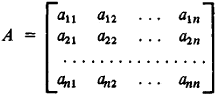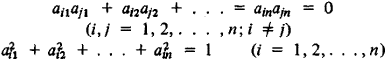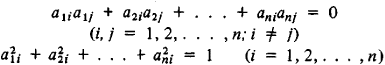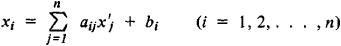Orthogonal Matrix

(redirected from Orthogonal matrices)
Also found in: Dictionary.

orthogonal matrix

[ȯr′thäg·ən·əl ′mā·triks]
(mathematics)
A matrix whose inverse and transpose are identical.

Orthogonal Matrix

An orthogonal matrix of order n is a matrixwhose product with the transpose A′ gives the identity matrix, that is, AA′ = E and AA = E. The elements of an orthogonal matrix satisfy the relationsor the equivalent relationsThe determinant ǀAǀ of an orthogonal matrix is equal to +1 or – 1. The product of two orthogonal matrices is an orthogonal matrix. All orthogonal matrices of order n form, with respect to the operation of multiplication, a group called the orthogonal group. In conversion from one rectangular coordinate system to another, the coefficients aij in the coordinate transformation equationsform an orthogonal matrix.

References in periodicals archive ?
The proof shows that (2.1) is a generalization of (2.2) by replacing the orthogonal matrices by weighted orthogonal matrices.
is, the set of [m.sub.1] x [m.sub.2] column orthogonal matrices is written as [mathematical expression not reproducible].
The only difference between OTSA and TSA or between ODTSA and DTSA is that U and V are constrained to orthogonal matrices in OTSA and ODTSA.
For given symmetric orthogonal matrices [R.sub.3] [member of] [MATHEMATICAL EXPRESSION NOT REPRODUCIBLE IN ASCII], [R.sub.4] [member of] [MATHEMATICAL EXPRESSION NOT REPRODUCIBLE IN ASCII], [R.sub.5] [member of] [MATHEMATICAL EXPRESSION NOT REPRODUCIBLE IN ASCII] and [S.sub.5] [member of] [MATHEMATICAL EXPRESSION NOT REPRODUCIBLE IN ASCII], then matrix equation (2) is solvable if and only if the following matrix equations are consistent, namely
[v.sub.N]) can be obtained on the basis of singular value decomposition of the matrix [[PHI].sup.[summation]] (Low et al, 1986): [[PHI].sup.[summation]] = [U.sub.[PHI]] x [V.sub.[PHI]], where [U.sub.[PHI]] and [V.sub.[PHI]] are orthogonal matrices, [MATHEMATICAL EXPRESSION NOT REPRODUCIBLE IN ASCII], and are singular values of [[PHI].sup.[summation]].
However the optimal approximation solution to a given matrix pair ([G.sup.*.sub.1], [G.sup.*.sub.2]) cannot be obtained in the corresponding solution set, and the difficulty is due to that the invariance of the Frobenius norm only holds for orthogonal matrices, but does not hold non-singular matrices that appear in CCD used in .
Topics include orthogonal matrices and wireless communications, probabilistic expectations on unstructured spaces, higher order necessary conditions in smooth constrained optimization, Hamiltonian paths and hyperbolic patterns, fair allocation methods for coalition games, sums-of-squares formulas, product-free subsets of groups, generalizations of product- free subsets, and vertex algebras and twisted bialgebras.
For example: Lecturing on "orthogonal matrices" which were introduced by the French mathematician Charles Hermite in 1854 we went further back to 1770 when Euler for the first time considered a system of linear equations in which an orthogonal matrix was used implicitly without knowing anything about matrices in general or orthogonal matrices in particular.
 Ann Lee: Secondary symmetric, secondary skew symmetric, secondary orthogonal matrices; Period Math.
As one might gather from this result, the set of all n x n orthogonal matrices plays a central role in our analysis.
where U (mxm) and V (nxn) are square, orthogonal matrices and [SIGMA] is a diagonal matrix mxn of singular values ([sigma]i j = 0 if i [not equal to] j and [sigma]11 [greater than or equal to] [sigma]22 [greater than or equal to] ...

Site: Follow: Share:
Open / Close